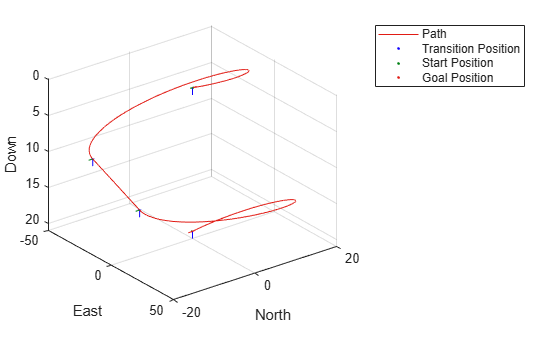uavDubinsPathSegment

Dubins path segment connecting two poses of UAV

Description

The uavDubinsPathSegment object holds information for a Dubins path segment that connects start and goal poses of a UAV as a sequence of motions in the north-east-down coordinate system.

The motion options are:

• Straight

• Left turn (counterclockwise)

• Right turn (clockwise)

• Helix left turn (counterclockwise)

• Helix right turn (clockwise)

• No motion

The turn direction is defined as viewed from the top of the UAV. Helical motions are used to ascend or descend.

Creation

Description

To generate a uavDubinsPathSegment object, use the connect function with a uavDubinsConnection object:

pathSegObj = connect(connectionObj,start,goal) connects the start and goal poses using the specified uavDubinsConnection object. The start and goal inputs set the value of the properties StartPose and GoalPose, respectively.

To specifically define a path segment:

pathSegObj = uavDubinsPathSegment(connectionObj,start,goal) creates a Dubins path segment to connect start and goal poses of a UAV. The uavDubinsConnection object provides the minimum turning radius and flight path angle. It internally computes the optimal path and assigns it to pathSegObj.

example

pathSegObj = uavDubinsPathSegment(connectionObj,start,goal,motionTypes) creates a Dubins path segment to connect start and goal poses of a UAV with the given motionTypes. The motionTypes input sets the value of the MotionTypes property.

pathSegObj = uavDubinsPathSegment(start,goal,flightPathAngle,airSpeed,minTurningRadius,helixRadius,motionTypes,motionLengths) creates a Dubins path segment to connect start and goal poses of a UAV by explicitly specifying all the parameters. The input values are set to their corresponding properties in the object.

Properties

expand all

Initial pose of the UAV at the start of the path segment, specified as a four-element numeric vector [x, y, z, headingAngle].

Data Types: double

Goal pose of the UAV at the end of the path segment, specified as a four-element numeric vector [x, y, z, headingAngle].

Data Types: double

Minimum turning radius of the UAV, specified as a positive numeric scalar in meters. This value corresponds to the radius of the circle at the maximum roll angle and a constant airspeed of the UAV.

Data Types: double

Helical path radius of the UAV, specified as a positive numeric scalar in meters.

Data Types: double

Flight path angle of the UAV to reach the goal altitude, specified as a positive numeric scalar in radians.

Data Types: double

Airspeed of the UAV, specified as a positive numeric scalar in m/s.

Data Types: double

Length of each motion in the path segment, specified as a four-element numeric vector in meters. Each motion length corresponds to a motion type specified in the MotionTypes property.

Data Types: double

Type of each motion in the path segment, specified as a three-element string cell array.

Motion TypeDescription
"S"

Straight

"L"

Left turn (counterclockwise)

"R"

Right turn (clockwise)

"Hl"

Helix left turn (counterclockwise)

"Hr"

Helix right turn (clockwise)

"N"

No motion

Note

The no motion segment "N" is used as a filler at the end when only three path segments are needed.

Each motion type corresponds to a motion length specified in the MotionLengths property.

For UAV Dubins connections, the available path types are: {'LSLN'} {'LSRN'} {'RSLN'} {'RSRN'} {'RLRN'} {'LRLN'} {'HlLSL'} {'HlLSR'} {'HrRSL'} {'HrRSR'} {'HrRLR'} {'HlLRL'} {'LSLHl'} {'LSRHr'} {'RSLHl'} {'RSRHr'} {'RLRHr'} {'LRLHl'} {'LRSL'} {'LRSR'} {'LRLR'} {'RLSR'} {'RLRL'} {'RLSL'} {'LSRL'} {'RSRL'} {'LSLR'} {'RSLR'}.

Example: {'L','R','L','N'}

Data Types: cell

Length of the path segment or the flight path, specified as a positive numeric scalar in meters. This length is the sum of the elements in the MotionLengths vector.

Data Types: double

Object Functions

 interpolate Interpolate poses along UAV Dubins path segment show Visualize UAV Dubins path segment

Examples

collapse all

This example shows how to calculate a UAV Dubins path segment and connect poses using the uavDubinsConnection object for a specified motion type.

Create a uavDubinsConnection object.

connectionObj = uavDubinsConnection;

Define start and goal poses as [x, y, z, headingAngle] vectors.

startPose = [0 0 0 0]; % [meters, meters, meters, radians]
goalPose = [0 0 20 pi];

Calculate a valid path segment and connect the poses for a specified motion type.

pathSegObj = uavDubinsPathSegment(connectionObj,startPose,goalPose,{'L','S','L','N'});

Show the generated path.

show(pathSegObj)Verify the motion type of the returned path segment.

fprintf('Motion Type: %s\n',strjoin(pathSegObj.MotionTypes));
Motion Type: L S L N

Extended Capabilities

C/C++ Code GenerationGenerate C and C++ code using MATLAB® Coder™.